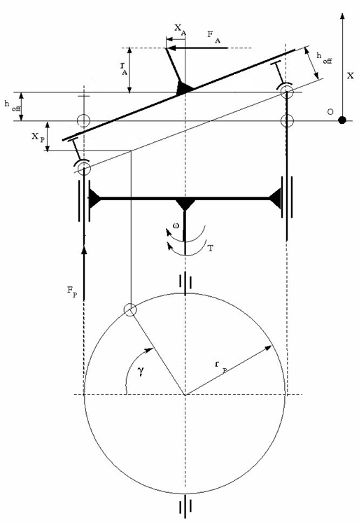# Swash Plate

Swash plate in a single-piston, isothermal axial piston pump

• Library:
• Simscape / Fluids / Isothermal Liquid / Pumps & Motors / Auxiliary Components

•## Description

The Swash Plate block models a single, piston-actuated swash plate within an axial-piston pump. It translates linear actuation into rotational motion that, when connected to the Valve Plate Orifice (IL) block, periodically connects the piston with the pump intake and discharge. You can connect multiple swash plate blocks to multiple pistons.

Schematic of a Swash Plate Within an Axial-Piston Pump Unit### Force Balance

The plate is in dynamic equilibrium if the net work by the piston, actuator, and plate is zero:

`${F}_{P}{\delta }_{P}+{F}_{A}{\delta }_{A}+\left(\tau -{\tau }_{res}\right){\delta }_{y}=0,$`

where:

• FPδP is the work done due to piston displacement.

• FAδA is the work done due to actuator displacement.

• (τ-τresy is the work done due to the net plate torque tangent to plate motion.

The plate torque is:

`$\tau =-\frac{{r}_{P}}{{r}_{A}}{x}_{A}{F}_{P}\mathrm{sin}\gamma +{\tau }_{res},$`

where:

• rP is the Cylinder block pitch radius.

• rA is the Swash plate actuator arm.

• XA is the actuator displacement.

• FP is the piston force.

• γ is the plate angular displacement.

The viscous friction torque, or the resistance to motion due to plate-piston contact, is:

`${\tau }_{res}={\mu }_{viscous}\omega {r}_{P}^{2},$`

where:

• μviscous is the Viscous friction coefficient.

• ω is the rotor angular velocity.

### Piston Dynamics

The piston displacement, x, is proportional to the actuator displacement:

`$x=-{h}_{off}\left(\sqrt{1+\frac{{x}_{A}^{2}}{{r}_{A}^{2}}}-1\right)-\frac{{r}_{P}}{{r}_{A}}{x}_{A}\mathrm{cos}\gamma ,$`

where hoff is the Piston offset from the plate. Displacement is positive if it moves toward the cylinder block.

The piston velocity is:

`${V}_{P}=\frac{{r}_{P}}{{r}_{A}}{x}_{A}\omega \mathrm{sin}\left(\gamma \right)-\frac{{r}_{P}}{{r}_{A}}{V}_{A}\mathrm{cos}\gamma -\frac{{h}_{off}}{{r}_{A}^{2}}\frac{{x}_{A}{V}_{A}}{\sqrt{1+\frac{{x}_{A}^{2}}{{r}_{A}^{2}}}},$`

where VA is the actuator velocity.

### Assumptions and Limitations

This block accounts for the effect of viscous friction between the piston and plate, but neglects plate and fluid inertia.

Note

This reference page is for the Isothermal Liquid library block. For the Hydraulics (Isothermal) Liquid library block, see Swash Plate.

## Ports

### Conserving

expand all

Swash plate actuator velocity and force.

Piston end velocity and force.

Drive shaft angular velocity and torque. Rotation is relative to the environment.

## Parameters

expand all

Length of fully extended actuator arm.

Pitch radius of the rotating cylinder.

Piston offset when the actuator is in neutral position. A positive, nonzero value indicates a partially retracted piston. A negative, nonzero value indicates an extended piston that remains within the cylinder block during an initial actuator displacement away from the cylinder.

Coefficient used in the equation for torque due to viscous friction.

## Version History

Introduced in R2020a